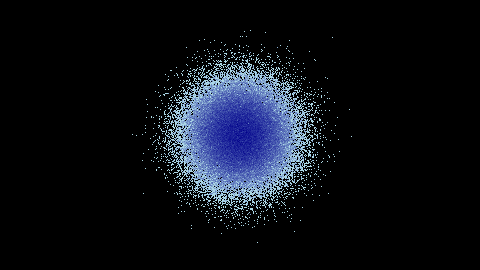# Backend: `datashader`

The `datashader` package is a high-performance graphics pipeline for visualizing very large quantities of data. For further details, please consult the documentation of datashader.

```import numpy as np
import pandas as pd
from bewegung import Video

v = Video(width = 480, height = 270, seconds = 1.0)

@v.sequence()
class Foo:

@v.layer(
canvas = v.canvas(
x_range = (-9.6, 9.6),
y_range = (-5.4, 5.4),
)
)
def bar(self, canvas): # a datashader canvas object

points = 100000
df = pd.DataFrame(dict(
x = np.random.normal(size = points),
y = np.random.normal(size = points),
))

agg = canvas.points(df, 'x', 'y')
img = tf.shade(agg, cmap = ['lightblue', 'darkblue'], how = 'log')

return img # a datashader.transfer_functions.Image object

v.reset()
v.render_frame(v.time(0))
```Similar to `datashader.Canvas`, the function call `v.canvas(backend = 'datashader')` accepts the following additional keyword arguments:

• `plot_width`, width of the video by default

• `plot_height`, height of the video by default

• `x_range`, by default `None`

• `y_range`, by default `None`

• `x_axis_type`, by default `'linear'`

• `y_axis_type`, by default `'linear'`

• `width`, mapped to `plot_width`

• `height`, mapped to `plot_height`

Layer methods are expected to return `datashader.transfer_functions.Image` objects or, alternatively, Pillow Image objects.

Warning

If a `datashader.transfer_functions.Image` object is returned, `bewegung` will mirror the image along the x-axis, i.e. the y-axis will be flipped. This makes the output consistent with `Pillow` and `pycairo`, were the y-axis is positive downwards. The flip can be avoided by manually converting the image to a Pillow Image object before returning, i.e. `return img.as_pil()` in the above example.